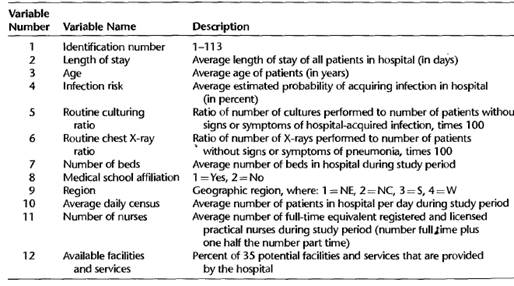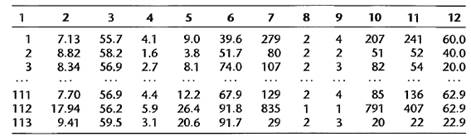# Refer to the SENIC data set in Appendix C.l. a. For each geographic region, regress infection… 1 answer below »

Refer to the SENIC data set in Appendix C.l.

a. For each geographic region, regress infection risk (Y) against the predictor variables age (X,), routine culturing ratio (X2), average daily census (X3 ) and available facilities and services (X4 ). Use first-order regression model (6.5) with four predictor variables. State the estimated regression functions.

b. Are the estimated regression functions similar for the four regions? Discuss

c. Calculate MSE and R2 for each region. Are these measures similar for the four regions? Discuss.

d. Obtain the residuals for each fitted model and prepare a box plot of the residuals for each fitted model Interpret the plots and state your findings.

Appendix C.l.

The primary objective of the Study on the Efficacy of Nosocomial Infection Control (SENIC Project) was to determine whether infection surveillance and control programs have reduced the rates of nosocomial (hospital-acquired) infection in United States hospitals. This data set consists of a random sample of 113 hospitals selected from the original 338 hospitals

surveyed. Each line of the data set has an identification number and provides information on 11 other variables for a single hospital. The data presented here are for the 1975-76 study period. The 12 variables are: